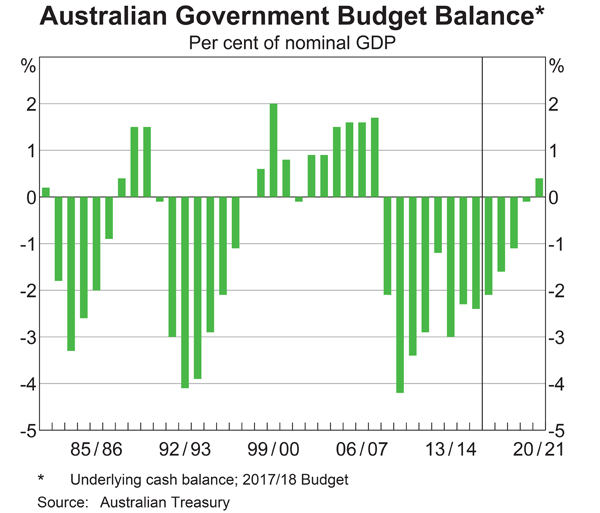# Fight Finance

#### CoursesTagsRandomAllRecentScores

The following equation is the Dividend Discount Model, also known as the 'Gordon Growth Model' or the 'Perpetuity with growth' equation.

$$P_{0} = \frac{C_1}{r_{\text{eff}} - g_{\text{eff}}}$$

What would you call the expression $C_1/P_0$?

Government bonds currently have a return of 5%. A stock has a beta of 2 and the market return is 7%. What is the expected return of the stock?

One formula for calculating a levered firm's free cash flow (FFCF, or CFFA) is to use net operating profit after tax (NOPAT).

\begin{aligned} FFCF &= NOPAT + Depr - CapEx -\Delta NWC \\ &= (Rev - COGS - Depr - FC)(1-t_c) + Depr - CapEx -\Delta NWC \\ \end{aligned} \\
Does this annual FFCF or the annual interest tax shield?

A European call option will mature in $T$ years with a strike price of $K$ dollars. The underlying asset has a price of $S$ dollars.

What is an expression for the payoff at maturity $(f_T)$ in dollars from having written (being short) the call option?

A stock just paid a dividend of $1. Future annual dividends are expected to grow by 2% pa. The next dividend of$1.02 (=1*(1+0.02)^1) will be in one year, and the year after that the dividend will be \$1.0404 (=1*(1+0.02)^2), and so on forever.

Its required total return is 10% pa. The total required return and growth rate of dividends are given as effective annual rates.

Calculate the current stock price.

A firm pays out all of its earnings as dividends. Because of this, the firm has no real growth in earnings, dividends or stock price since there is no re-investment back into the firm to buy new assets and make higher earnings. The dividend discount model is suitable to value this company.

The firm's revenues and costs are expected to increase by inflation in the foreseeable future. The firm has no debt. It operates in the services industry and has few physical assets so there is negligible depreciation expense and negligible net working capital required.

Which of the following statements about this firm's PE ratio is NOT correct? The PE ratio should:

Note: The inverse of x is 1/x.

Which of the following statements about standard statistical mathematics notation is NOT correct?

A stock has a beta of 1.5. The market's expected total return is 10% pa and the risk free rate is 5% pa, both given as effective annual rates.

Over the last year, bad economic news was released showing a higher chance of recession. Over this time the share market fell by 1%. So $r_{m} = (P_{0} - P_{-1})/P_{-1} = -0.01$, where the current time is zero and one year ago is time -1. The risk free rate was unchanged.

What do you think was the stock's historical return over the last year, given as an effective annual rate?

Below is the Australian federal government’s budget balance as a percent of GDP.From 2009 to 2016 the Australian federal government has implemented:

Question 858  indirect security, intermediated finance, no explanation

Which of the following transactions involves an ‘indirect security’ using a ‘financial intermediary’?